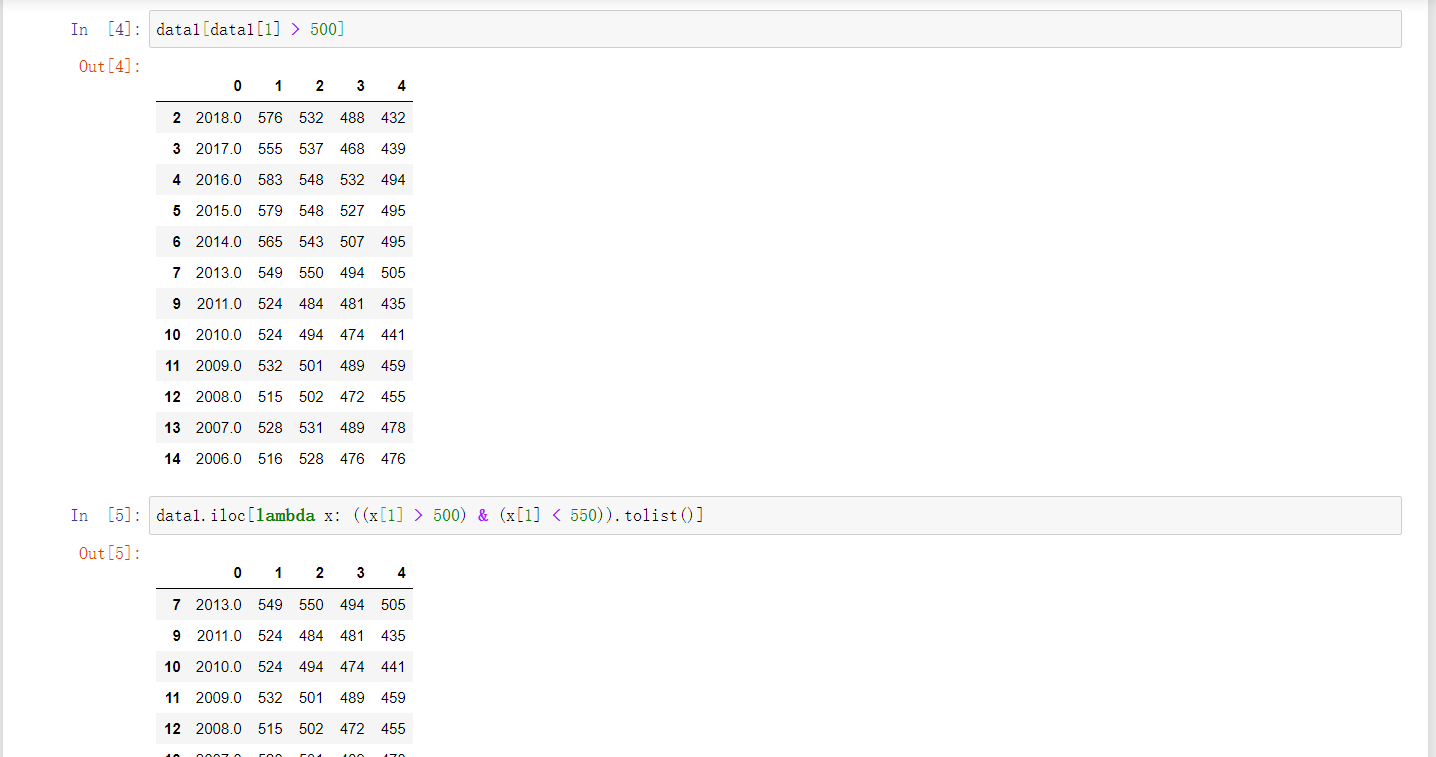4050 字

# 简介

Pandas是Python的一个数据分析包，最初于2008年4月开发，2009年底开源，目前由PyData开发团队继续开发和维护。它是为了解决数据分析任务而创建的基于Numpy的工具，纳入了大量库和一些标准的数据模型，提供了高效地操作大型数据集所需的工具和大量能使我们快速便捷地处理数据的函数和方法，最初被作为金融数据分析工具而开发出来，也为时间序列分析提供了很好的支持。

# 数据结构

## Series

Series是带标签的一维数组，与 Numpy中的维array类似，可以更有效的使用内存，提高运算效率，可存储不同类型的数据。它又像是一个Python的dict类型，存在索引与元素是映射关系，若在创建时没有显式指定索引，则默认索引为[0:N-1]。

### 构造

Series的构造函数为：

pd.Series([data, index, dtype, name, copy, ...])

data 初始化数据，可为数组、列表、字典等
index 索引值必须唯一和散列，与数据长度相同
dtype 数据类型
name 设置名称
copy 复制数据

# 1.数组创建
ser = pd.Series([10, 7, -4, 1])

# 2.字典创建+指定索引
data = {"a":1.,"b":2,"c":3,"d":4}
ser = pd.Series(data,index=["d","c","b","a"])

# 3.常量创建
ser = pd.Series(pd.Series(5,index=[1,2,3,4,5]))

### 成员变量

values 以数组方式获取Series的元素值
index 以数组方式获取Series的元素索引
name 获取values的name（需额外指定）
index.name 获取index的name（需额外指定）
dtype 获取Series数据类型
array 以数组方式获取Series的值，与values的区别在于array返回的是PandasArray()数据结构

## DataFrame

DataFrame是带标签且大小可变的二维表格型数据结构，可以将DataFrame理解为Series的容器。DataFrame由按一定顺序排列的多列数据组成，设计初衷是将Series的使用场景从一维拓展到多维，它既有行索引，也有列索引。

DataFrame数据是以一个或多个二维块存放的，而不是别的一维数据结构

### 构造

DataFrame的构造函数：

pd.DataFrame(data=None, index=None, columns=None, dtype=None, copy=False)

data 数据采取各种形式，如:ndarray，series，map，lists，dict，constant和另一个DataFrame
index 行索引，缺省值np.arrange(n)
columns 列索引，缺省值np.arrange(n)
dtype 数据类型
name 设置名称
copy 复制数据

# 1. 单个列表+指定行索引
data = [1,2,3,4,5]
df = pd.DataFrame(data, index=['1','2','3','4', '5'])

# 2. 列表套列表+指定列索引
data = [['Alex',10],['Bob',12],['Clarke',13]]
df = pd.DataFrame(data,columns=["name","age"])

# 3. 字典（键为列索引）
data = {'Name':['Tom', 'Jack', 'Steve', 'Ricky'],'Age':[28,34,29,42]}
df = pd.DataFrame(data)

# 4. 字典列表
data = [{'a': 1, 'b': 2},{'a': 5, 'b': 10, 'c': 20}]  # 字典键默认为列名,没有值得为NaN
df = pd.DataFrame(data,index=["first","second"])  # 自定义行索引

# 5. numpy
pd.DataFrame(np.random.randint(60,100,size=(3,4)))  # 60-100随机选择,3行4列

### 成员变量

values 取出所有值
index 以数组方式返回行标签
columns 以数组方式返回列标签
axes 以二维数组方式返回行标签与列标签
T 以数组方式返回行列转置后的DataFrame，类似于矩阵
shape 当前表是几行几列

# 索引

Pandas各种数据类型的索引使用方法基本通用，本节使用DataFrame为例

## 切片索引

1. 选中特定行：data[m:n:step]（范围[m,n)
2. 选择特定列: data['col1']data[['col1','col2']]
3. 选择特定区域：data[row_index][col_index]

## loc索引

loc索引使用格式为data.loc[row_index][col_index]data.loc[row_index, col_index]，主要有以下几种情况（与上面大致相同）：

1. 选中特定行：data.loc[m:n:step]（范围[m,n)
2. 选择特定列: data.loc[['col1','col2']]
3. 选择特定区域：data.loc[row_index][col_index]

## iloc索引

iloc索引使用格式为data.iloc[row_index][col_index]data.iloc[row_index, col_index]，主要有以下几种情况（与上面大致相同）：

1. 选中特定行：data.iloc[m:n:step]（范围[m,n)
2. 选择特定列: data.iloc[[col1,col2]]
3. 选择特定区域：data.iloc[row_index][col_index]

loc row_indexcol_index只能使用索引名

iloc row_indexcol_index只能使用序号索引

## 条件索引1

1. 直接条件：data1[data1 > 500]（文科一本分数线大于500的行）
2. lambda函数：data1.iloc[lambda x: ((x > 500) & (x < 550)).tolist()]（文科一本分数线大于500小于550的行）条件索引

## 层次索引2

### 构造

1. 隐式构造
Python data.columns = [['一本分数线','一本分数线','二本分数线','二本分数线'],['文科','理科','文科','理科']]
2. 显式构造

# 1. 从tuple
multiIndex = pd.MultiIndex.from_tuples([('一本分数线','文科'),('一本分数线','理科'),('二本分数线','文科'),('二本分数线','文科')])
# 2. 从list
multiIndex = pd.MultiIndex.from_arrays([['一本分数线','一本分数线','二本分数线','二本分数线'],['文科','理科','文科','理科']])
# 3. 从product
multiIndex = pd.MultiIndex.from_product([['一本分数线','二本分数线'],['文科','理科']])
data.columns = multiIndex

### 访问

data[1:4]['一本分数线','文科']
data.loc[1:4,['一本分数线','文科']]

# 通用方法

df.info() 打印二维数组的信息，包含行列标签，内存大小，
df.head(i)/df.tail(i) 显示前/后i行数据，以行为单位
df.describe() 查看数据值列的汇总统计
df.drop() 删除指定位置的数据
df1+df2 在运算中自动对齐不同索引的数据，如果索引不对应，则补NaN
ser.reindex() 重设索引，该函数会创建一个新的对象
ser.unique() 对series元素进行去重
ser.isnull()/ser.isnull() 缺失的数据返回的布尔值为True/False

# 数据读写

## 通用参数

filepath 文件存储路径，可以用r""进行非转义限定
encoding 指定数据编码
sep 指定分割符
header 指定第一行是否是列名：0(数据第一行为列名）、None（表明数据没有列名）。常与names搭配使用
names 指定列名
usecols 指定读取的列名
index_col 指定哪几列作为索引
skiprows 跳过行数
nrows 读取行数

# 统计方法

df.count() 非 NA 值的数量
df.describe() 针对 Series 或 DF 的列计算汇总统计
df.min()/df.max() 最小值和最大值
df.argmin()/df.argmax() 最小值和最大值的索引位置（整数）
df.idxmin()/df.idxmax() 最小值和最大值的索引值
df.quantile() 样本分位数（0 到 1）
df.sum() 求和
df.mean() 均值
df.median() 中位数
df.mad() 根据均值计算平均绝对离差
df.var() 方差
df.std() 标准差
df.skew() 样本值的偏度（三阶矩）
df.kurt() 样本值的峰度（四阶矩）
df.cumsum() 样本值的累计和
df.cummin()/df.cummax() 样本值的累计最大值和累计最小值
df.cumprod() 样本值的累计积
df.diff() 计算一阶差分（对时间序列很有用）
df.pct_change() 计算百分数变化

# 遍历与排序

## 遍历

df.iterrows() 将DataFrame的每一行迭代为(index, Series)对，可以通过row[name]对元素进行访问
df.iteritems() 将DataFrame的每一列迭代为(列名, Series)对，可以通过row[index]对元素进行访问
df.itertuples() 将DataFrame的每一行迭代为元祖，可以通过row[name]对元素进行访问，比iterrows()效率高

## 排序

df.sort_index() 将按行/列索引排序
df.sort_values() 按指定值排序

# 缺失值处理

df.dropna() 删除缺失值
df.isna()/df.notna() 判断缺失值
df.fillna()/df.interpolate() 判断缺失值

# 参考

1. SNII_629. [Pandas]数据选取/数据切片. CSDN. [2018-10-09] [return]
2. 你好，小帝. pandas层次化索引. 博客园. [2017-10-26] [return]